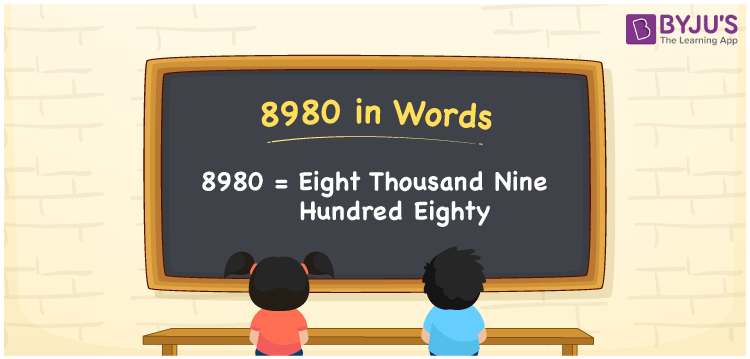# 8980 in Words

We can write 8980 in words as Eight thousand nine hundred eighty. It is possible to convert the number 8980 to words with the help of a place value chart. Also, we know that 8980 is a cardinal number since it indicates a specific amount or quantity. If you won a reward worth Rs. 8980 in a competition, you can say, “I won a reward worth Eight thousand nine hundred eighty rupees in the competition”.

 8980 in words Eight thousand nine hundred eighty Eight thousand nine hundred eighty in Numbers 8980

## 8980 in English Words

We generally express numbers in words using the English alphabet. Thus, we spell 8980 in English as “Eight thousand nine hundred eighty”.## How to Write 8980 in Words?

In this section, you will learn how to convert the number 8980 into words using a place value chart. The below table shows place values for all digits of 8980.

 Thousands Hundreds Tens Ones 8 9 8 0

Here, ones = 0, tens = 8, hundreds = 9, and thousands = 8.

Thus, we can write the expanded form of these digits as:

8 × Thousand + 9 × Hundred + 8 × Ten + 0 × One

= 8 × 1000 + 9 × 100 + 8 × 10 + 0 × 1

= 8000 + 900 + 80

= Eight thousand + Nine hundred + Eighty

= Eight thousand nine hundred eighty

Therefore, 8980 in words is written as Eight thousand nine hundred eighty.

8980 is a natural number that precedes 8981 and succeeds 8979.

8980 in words – Eight thousand nine hundred eighty

Is 8980 an odd number? – No

Is 8980 an even number? – Yes

Is 8980 a prime number? – No

Is 8980 a composite number? – Yes

Is 8980 a perfect square number? – No

Is 8980 a perfect cube number? – No

## Frequently Asked Questions on 8980 in Words

Q1

### How do you write 8980 in words?

We can write 8980 in words as Eight thousand nine hundred eighty.
Q2

### What is the number name of 8980?

The number name of 8980 is “Eight thousand nine hundred eighty”.
Q3

### How to write Rs. 8980 in words on a cheque?

On a cheque, we generally write an amount of Rs. 8980 in words as Eight thousand nine hundred eighty rupees only.